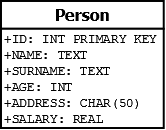Open In App

# SQL using C/C++ and SQLite

Before we go on with this tutorial, we need to follow the SQLITE3 installation procedure that can be easily found here. At the same time it is required a basic knowledge of SQL.

We will show the following operations:

• Database Connection/Creation
• Create Table
• Insert
• Delete
• Select

For the sake of simplicity, let’s use a simple database made of just one table.Database Connection/Creation of Table

In this snippet, we will use two routines contained in sqlite3.h library.

```- sqlite3_open(const char *filename, sqlite3 **ppDb)
- sqlite3_close(sqlite3 *ppDb)
```

The Compiling is executed by the add of command -l sqlite3 .

 `#include ``#include `` ` `int` `main(``int` `argc, ``char``** argv)``{``    ``sqlite3* DB;``    ``int` `exit` `= 0;``    ``exit` `= sqlite3_open(``"example.db"``, &DB);`` ` `    ``if` `(``exit``) {``        ``std::cerr << ``"Error open DB "` `<< sqlite3_errmsg(DB) << std::endl;``        ``return` `(-1);``    ``}``    ``else``        ``std::cout << ``"Opened Database Successfully!"` `<< std::endl;``    ``sqlite3_close(DB);``    ``return` `(0);``}`

Output:

```\$ g++ createDB.cpp -l sqlite3
\$ ./a.out

Opened Database Successfully!

\$ ls
create.cpp
a.out
example.db
```

The first routine returns an integer: if the integer is equal to 0, it succeeded. If the database doesn’t exist yet, it is going to be created in the same directory where the process was executed.
The second one just closes the connection previously opened by SQLITE3_OPEN().
All prepared statement associated with the connection should be finalized prior to closing the connection.

Create Table

In this snippet we will use the routine:

```- sqlite3_exec(sqlite3*, const char *sql, sqlite_callback, void *data, char **errmsg)
```

 `#include ``#include `` ` `int` `main(``int` `argc, ``char``** argv)``{``    ``sqlite3* DB;``    ``std::string sql = ``"CREATE TABLE PERSON("``                      ``"ID INT PRIMARY KEY     NOT NULL, "``                      ``"NAME           TEXT    NOT NULL, "``                      ``"SURNAME          TEXT     NOT NULL, "``                      ``"AGE            INT     NOT NULL, "``                      ``"ADDRESS        CHAR(50), "``                      ``"SALARY         REAL );"``;``    ``int` `exit` `= 0;``    ``exit` `= sqlite3_open(``"example.db"``, &DB);``    ``char``* messaggeError;``    ``exit` `= sqlite3_exec(DB, sql.c_str(), NULL, 0, &messaggeError);`` ` `    ``if` `(``exit` `!= SQLITE_OK) {``        ``std::cerr << ``"Error Create Table"` `<< std::endl;``        ``sqlite3_free(messaggeError);``    ``}``    ``else``        ``std::cout << ``"Table created Successfully"` `<< std::endl;``    ``sqlite3_close(DB);``    ``return` `(0);``}`

Output:

```\$ g++ createTable.cpp -l sqlite3
\$ ./a.out

Table created Successfully

```

This routine returns an integer: if the integer is equal to the SQLITE_OK macro, everything worked well.
For this example, the controls about open of Database are omitted, that are written in the past code.

Insert and Delete

We will use the SQLITE3_EXEC() routine for Insert as well. Procedure and checks are similar to the previous one. There is just one difference in the string that we use with the SQLITE3_EXEC().

 `#include ``#include ``#include `` ` `using` `namespace` `std;`` ` `static` `int` `callback(``void``* data, ``int` `argc, ``char``** argv, ``char``** azColName)``{``    ``int` `i;``    ``fprintf``(stderr, ``"%s: "``, (``const` `char``*)data);`` ` `    ``for` `(i = 0; i < argc; i++) {``        ``printf``(``"%s = %s\n"``, azColName[i], argv[i] ? argv[i] : ``"NULL"``);``    ``}`` ` `    ``printf``(``"\n"``);``    ``return` `0;``}`` ` `int` `main(``int` `argc, ``char``** argv)``{``    ``sqlite3* DB;``    ``char``* messaggeError;``    ``int` `exit` `= sqlite3_open(``"example.db"``, &DB);``    ``string query = ``"SELECT * FROM PERSON;"``;`` ` `    ``cout << ``"STATE OF TABLE BEFORE INSERT"` `<< endl;`` ` `    ``sqlite3_exec(DB, query.c_str(), callback, NULL, NULL);`` ` `    ``string sql(``"INSERT INTO PERSON VALUES(1, 'STEVE', 'GATES', 30, 'PALO ALTO', 1000.0);"``               ``"INSERT INTO PERSON VALUES(2, 'BILL', 'ALLEN', 20, 'SEATTLE', 300.22);"``               ``"INSERT INTO PERSON VALUES(3, 'PAUL', 'JOBS', 24, 'SEATTLE', 9900.0);"``);`` ` `    ``exit` `= sqlite3_exec(DB, sql.c_str(), NULL, 0, &messaggeError);``    ``if` `(``exit` `!= SQLITE_OK) {``        ``std::cerr << ``"Error Insert"` `<< std::endl;``        ``sqlite3_free(messaggeError);``    ``}``    ``else``        ``std::cout << ``"Records created Successfully!"` `<< std::endl;`` ` `    ``cout << ``"STATE OF TABLE AFTER INSERT"` `<< endl;`` ` `    ``sqlite3_exec(DB, query.c_str(), callback, NULL, NULL);`` ` `    ``sql = ``"DELETE FROM PERSON WHERE ID = 2;"``;``    ``exit` `= sqlite3_exec(DB, sql.c_str(), NULL, 0, &messaggeError);``    ``if` `(``exit` `!= SQLITE_OK) {``        ``std::cerr << ``"Error DELETE"` `<< std::endl;``        ``sqlite3_free(messaggeError);``    ``}``    ``else``        ``std::cout << ``"Record deleted Successfully!"` `<< std::endl;`` ` `    ``cout << ``"STATE OF TABLE AFTER DELETE OF ELEMENT"` `<< endl;``    ``sqlite3_exec(DB, query.c_str(), callback, NULL, NULL);`` ` `    ``sqlite3_close(DB);``    ``return` `(0);``}`

Output:

```\$ g++ insertDelete.cpp -l sqlite3
\$ ./a.out
STATE OF TABLE BEFORE INSERT
Records created Successfully!
STATE OF TABLE AFTER INSERT
ID = 1
NAME = STEVE
SURNAME = GATES
AGE = 30
SALARY = 1000.0

ID = 2
NAME = BILL
SURNAME = ALLEN
AGE = 20
SALARY = 300.22

ID = 3
NAME = PAUL
SURNAME = JOBS
AGE = 24
SALARY = 9900.0

Record deleted Successfully!
STATE OF TABLE AFTER DELETE OF ELEMENT
ID = 1
NAME = STEVE
SURNAME = GATES
AGE = 30
SALARY = 1000.0

ID = 3
NAME = PAUL
SURNAME = JOBS
AGE = 24
SALARY = 9900.0

```

Select

Before we go on showing the Select operation, that we might just as easily consider the most important of all the tutorial, let’s have a look at the Callback prototype, that we are going to use in our example.
This operation lets us to get an output from the Select operation:

```typedef int (*sqlite3_callback)(
void*,    /* Data provided in the 4th argument of sqlite3_exec() */
int,      /* The number of columns in row */
char**,   /* An array of strings representing fields in the row */
char**    /* An array of strings representing column names */
);
```

Now, we will use the Callback function into the Select as shown in the next snippet:

 `#include ``#include `` ` `using` `namespace` `std;`` ` `static` `int` `callback(``void``* data, ``int` `argc, ``char``** argv, ``char``** azColName)``{``    ``int` `i;``    ``fprintf``(stderr, ``"%s: "``, (``const` `char``*)data);`` ` `    ``for` `(i = 0; i < argc; i++) {``        ``printf``(``"%s = %s\n"``, azColName[i], argv[i] ? argv[i] : ``"NULL"``);``    ``}`` ` `    ``printf``(``"\n"``);``    ``return` `0;``}`` ` `int` `main(``int` `argc, ``char``** argv)``{``    ``sqlite3* DB;``    ``int` `exit` `= 0;``    ``exit` `= sqlite3_open(``"example.db"``, &DB);``    ``string data(``"CALLBACK FUNCTION"``);`` ` `    ``string sql(``"SELECT * FROM PERSON;"``);``    ``if` `(``exit``) {``        ``std::cerr << ``"Error open DB "` `<< sqlite3_errmsg(DB) << std::endl;``        ``return` `(-1);``    ``}``    ``else``        ``std::cout << ``"Opened Database Successfully!"` `<< std::endl;`` ` `    ``int` `rc = sqlite3_exec(DB, sql.c_str(), callback, (``void``*)data.c_str(), NULL);`` ` `    ``if` `(rc != SQLITE_OK)``        ``cerr << ``"Error SELECT"` `<< endl;``    ``else` `{``        ``cout << ``"Operation OK!"` `<< endl;``    ``}`` ` `    ``sqlite3_close(DB);``    ``return` `(0);``}`

Output:
\$ g++ select.cpp -l sqlite3
\$ ./a.out

```Opened Database Successfully!
RESULT OF SELECT
ID = 1
NAME = STEVE
SURNAME = GATES
AGE = 30
SALARY = 1000.0

ID = 3
NAME = PAUL
SURNAME = JOBS
AGE = 24